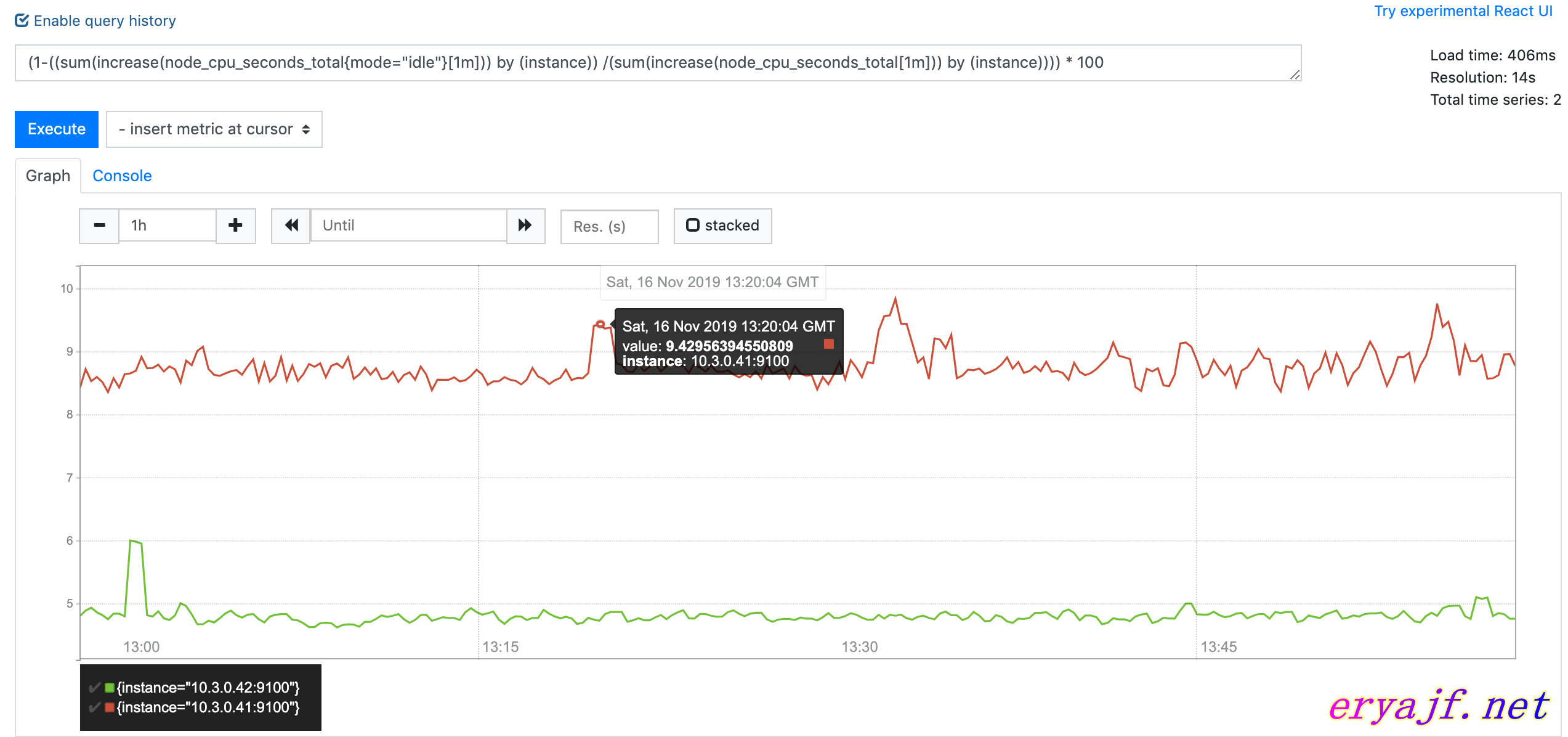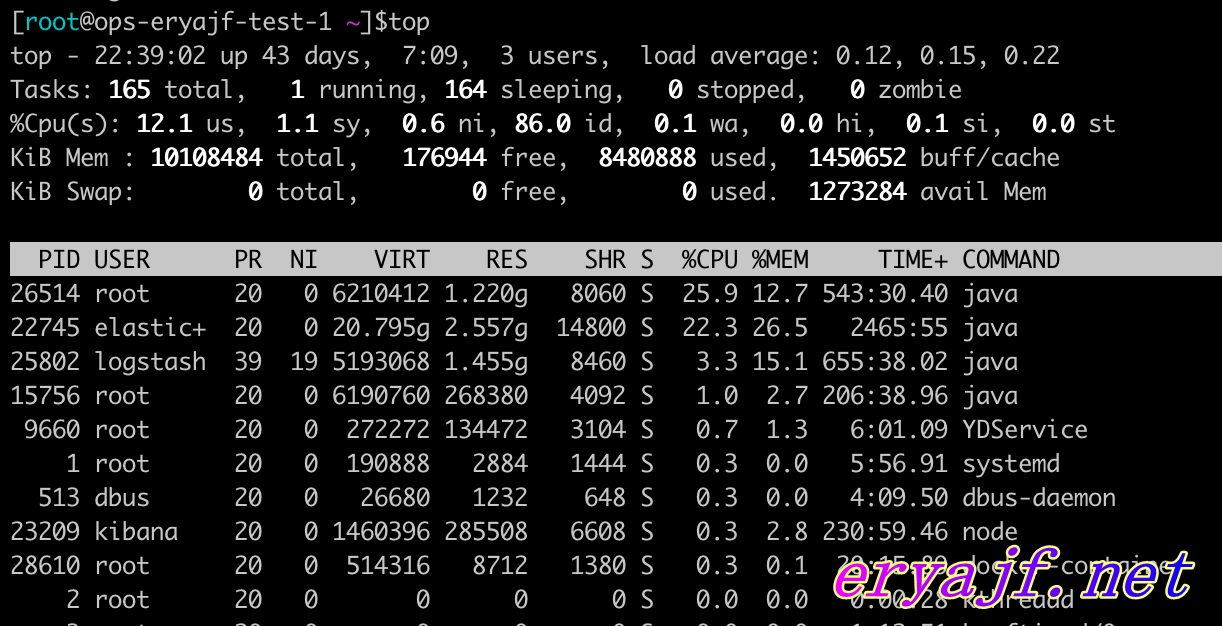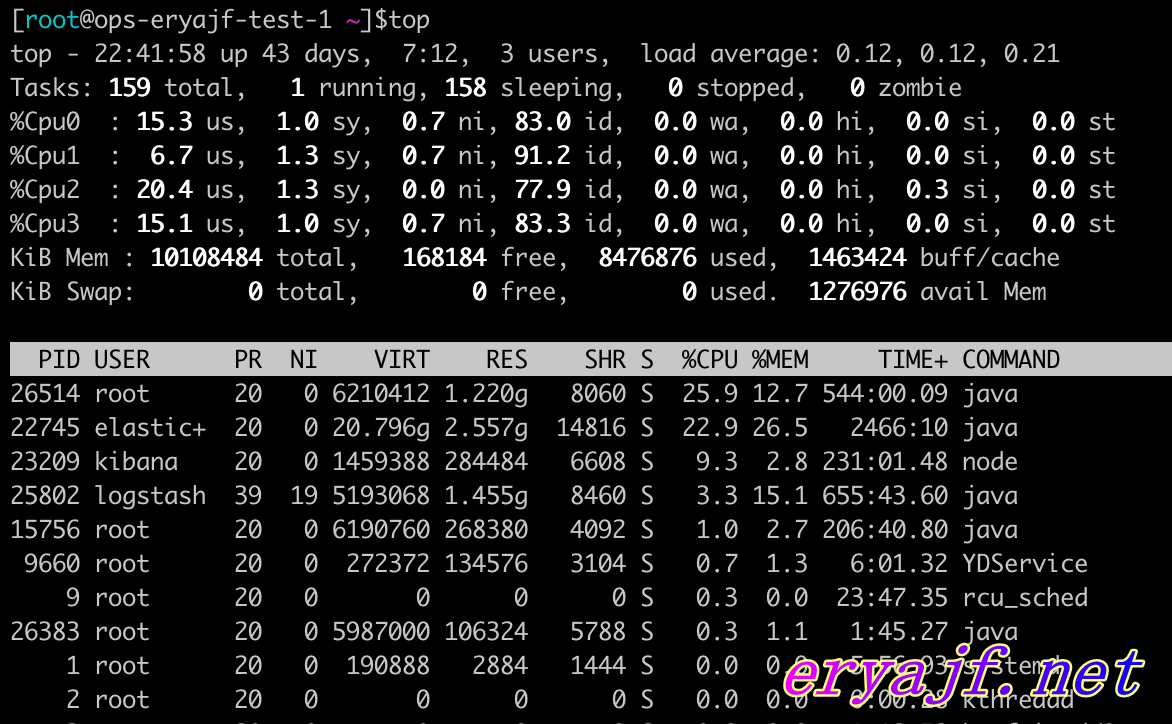• 对于注定会优秀的人来说，他所需要的，只是时间!
• 手懒得，必受贫穷，手勤的，必得富足----《圣经》
• 帮助别人，成就自己。愿君在本站能真正有所收获！
• 如果你在本站中发现任何问题，欢迎留言指正！
• 宝剑锋从磨砺出，梅花香自苦寒来！

# <六>Prometheus学习笔记–从CPU的获取来学习理解查询语句

1年前 (2020-02-05) 2447°C

## 1，引子

``````(1-((sum(increase(node_cpu_seconds_total{mode="idle"}[1m])) by (instance)) /(sum(increase(node_cpu_seconds_total[1m])) by (instance)))) * 100
``````## 2，关于CPU

### 1，问两个问题### 2，CPU详解

``````curl 127.0.0.1:9100/metrics
``````

``````# HELP node_cpu_seconds_total Seconds the cpus spent in each mode.
# TYPE node_cpu_seconds_total counter
node_cpu_seconds_total{cpu="0",mode="idle"} 3.5753192e+06
node_cpu_seconds_total{cpu="0",mode="iowait"} 2862.38
node_cpu_seconds_total{cpu="0",mode="irq"} 0
node_cpu_seconds_total{cpu="0",mode="nice"} 10430.32
node_cpu_seconds_total{cpu="0",mode="softirq"} 1593.13
node_cpu_seconds_total{cpu="0",mode="steal"} 0
node_cpu_seconds_total{cpu="0",mode="system"} 20133.98
node_cpu_seconds_total{cpu="0",mode="user"} 114790.89
node_cpu_seconds_total{cpu="1",mode="idle"} 3.53955659e+06
node_cpu_seconds_total{cpu="1",mode="iowait"} 3402
node_cpu_seconds_total{cpu="1",mode="irq"} 0
node_cpu_seconds_total{cpu="1",mode="nice"} 15018.42
node_cpu_seconds_total{cpu="1",mode="softirq"} 1084.01
node_cpu_seconds_total{cpu="1",mode="steal"} 0
node_cpu_seconds_total{cpu="1",mode="system"} 23992.78
node_cpu_seconds_total{cpu="1",mode="user"} 139793.89
node_cpu_seconds_total{cpu="2",mode="idle"} 3.50768181e+06
node_cpu_seconds_total{cpu="2",mode="iowait"} 3373.99
node_cpu_seconds_total{cpu="2",mode="irq"} 0
node_cpu_seconds_total{cpu="2",mode="nice"} 13949.32
node_cpu_seconds_total{cpu="2",mode="softirq"} 1047.97
node_cpu_seconds_total{cpu="2",mode="steal"} 0
node_cpu_seconds_total{cpu="2",mode="system"} 22868.3
node_cpu_seconds_total{cpu="2",mode="user"} 175018.57
node_cpu_seconds_total{cpu="3",mode="idle"} 3.54823306e+06
node_cpu_seconds_total{cpu="3",mode="iowait"} 3286.86
node_cpu_seconds_total{cpu="3",mode="irq"} 0
node_cpu_seconds_total{cpu="3",mode="nice"} 14471.86
node_cpu_seconds_total{cpu="3",mode="softirq"} 996.37
node_cpu_seconds_total{cpu="3",mode="steal"} 0
node_cpu_seconds_total{cpu="3",mode="system"} 22494.83
node_cpu_seconds_total{cpu="3",mode="user"} 135483.97
``````

• `user`(us)
表示用户态空间或者说是用户进程(running user space processes)使用CPU所耗费的时间。这是日常我们部署的应用所在的层面，最常见常用。
• `system`(sy)
表示内核态层级使用CPU所耗费的时间。分配内存、IO操作、创建子进程……都是内核操作。这也表明，当IO操作频繁时，System参数会很高。
• `steal`(st)
当运行在虚拟化环境中，花费在其它 OS 中的时间（基于虚拟机监视器 hypervisor 的调度）；可以理解成由于虚拟机调度器将 cpu 时间用于其它 OS 了，故当前 OS 无法使用 CPU 的时间。
• `softirq`(si)
从系统启动开始，累计到当前时刻，软中断时间
• `irq`(hi)
从系统启动开始，累计到当前时刻，硬中断时间
• `nice`(ni)
从系统启动开始，累计到当前时刻， 低优先级(低优先级意味着进程 nice 值小于 0)用户态的进程所占用的CPU时间
• `iowait`(wa)
从系统启动开始，累计到当前时刻，IO等待时间
• `idle`(id)
从系统启动开始，累计到当前时刻，除IO等待时间以外的其它等待时间，亦即空闲时间

``````total = user + nice + system + idle + iowait + irq + softirq + steal
``````

``````%us=(User time + Nice time)/total * 100%
%sy=(System time + Hard Irq time +SoftIRQ time)/total * 100%
%id=(Idle time)/total * 100%
%ni=(Nice time)/total * 100%
%wa=(Waiting time)/total * 100%
%hi=(Hard Irq time)/total * 100%
%si=(SoftIRQ time)/total * 100%
%st=(Steal time)/total * 100%
``````

## 3，再回首

``````(1-((sum(increase(node_cpu_seconds_total{mode="idle"}[1m])) by (instance)) /(sum(increase(node_cpu_seconds_total[1m])) by (instance)))) * 100
``````

• `1-((sum(increase(node_cpu_seconds_total{mode="idle"}[1m])) by (instance))`：这句话从内到外进行解析，后边计算的是1分钟内所有CPU的空闲时间，前边1减去这个时间，得出的就是正常使用的时间。
• `(sum(increase(node_cpu_seconds_total[1m])) by (instance)))`：这句话表达的意思更加简单，就是计算1分钟内，CPU的总时间。
• 剩下的部分，直白说就是 `使用时间` 除以 `总时间` 再乘以 `一百` 最终得出1分钟内CPU的使用率。[如果想支持本站，可支付宝赞助]• 自演自表

一生很短，
却又漫漫，
每个阶段
都应该有每个阶段的热爱。
爱，
就大胆争取，
爱，
就矢志不渝。
为爱出发，不失本心！
• 牧童遥指

• 接引即用

• 勿谓言之不预

本站的文章和资源来自互联网或者站长
的原创，按照 CC BY -NC -SA 3.0 CN
协议发布和共享，转载或引用本站文章
应遵循相同协议。如果有侵犯版权的资
源请尽快联系站长，我们会在24h内删
除有争议的资源。

既此勿忘：
学习，记录，分享。